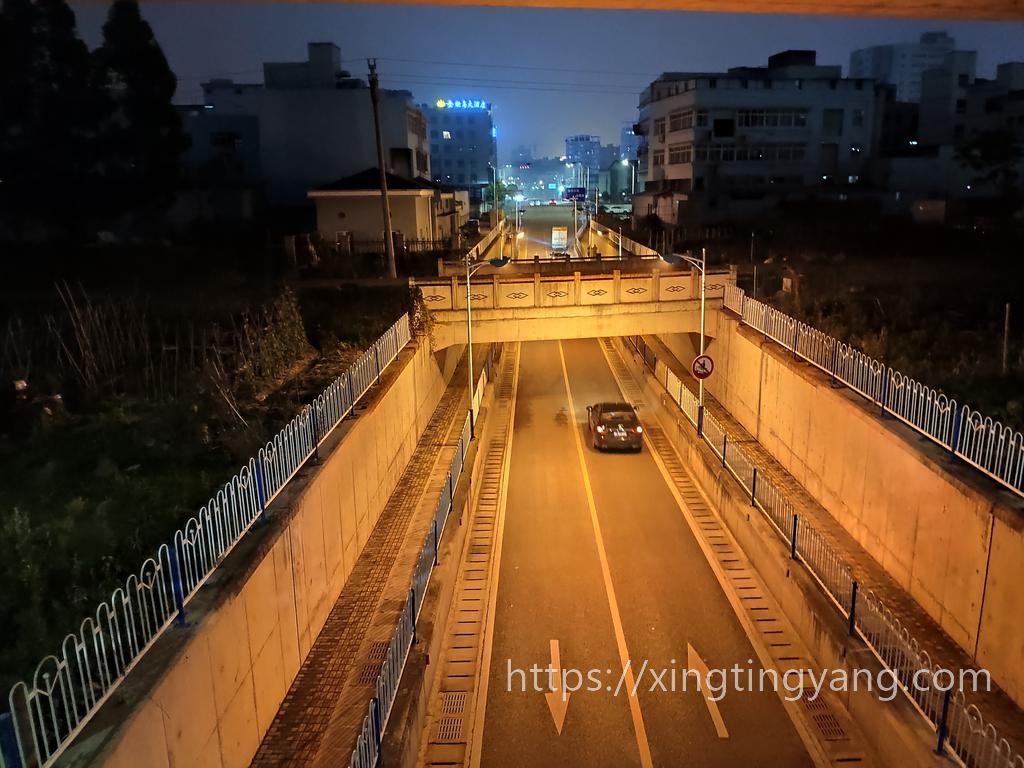# 2020/05/11 - 三角梅的花开了

PIL判断图片是否旋转的代码如下

# 判断是否图片需要旋转
try:
orientation = raw_image._getexif().get(274)
raw_image = raw_image.convert('RGBA')
if orientation == 3:
raw_image = raw_image.rotate(180, expand=True)
elif orientation == 6:
raw_image = raw_image.rotate(270, expand=True)
elif orientation == 8:
raw_image = raw_image.rotate(90, expand=True)
except:
raw_image = raw_image.convert('RGBA')